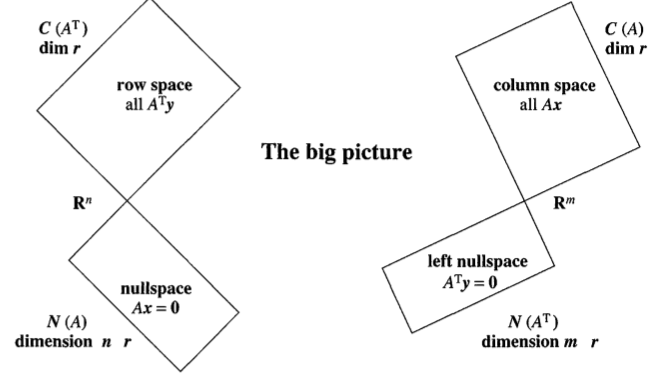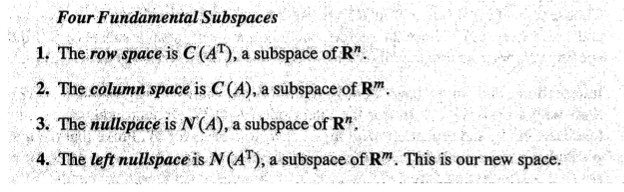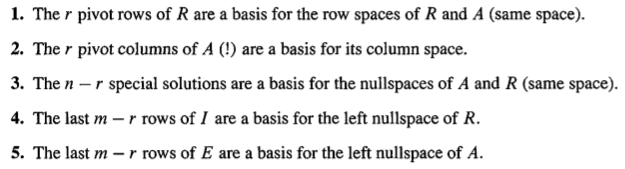Abstract: 四个向量空间的dimensions的一些性质
Keywords: Dimensions,Four Subspaces

# 四个子空间的维度

## 四个子空间(Four Subspaces)nullspace就是free columns的数量n-r，left nullspace就是free rows的数量m-r

### $R$

R是经过消元的矩阵，可以很容易的看出矩阵的rank，假设矩阵A是m by n的规模：
1) $N(R)$ 的dimensions是n-r
2) $C(R)$ 的dimensions是r
3) $C(R^T)$ 的dimensions是m-r

### $A$

1) A has the same row space as R.Same dimension r and same basis
2) The column space of A has dimension r.For every matrix this is essential.
3) A has the same nullspace as R.Same dimension n-r and same basis
4) The left nullspace of A(the nullspace of$A^T$) has dimension m-r

Fundamental Theorem of Linear Algebra ,Part 1
The column space and row space both have dimension r
The nullspaces have dimensions n-r and m-r

## conclusion0%